# Math in Focus Grade 7 Chapter 8 Lesson 8.1 Answer Key Recognizing Cylinders, Cones, Spheres, and Pyramids

This handy Math in Focus Grade 7 Workbook Answer Key Chapter 8 Lesson 8.1 Recognizing Cylinders, Cones, Spheres, and Pyramids detailed solutions for the textbook questions.

## Math in Focus Grade 7 Course 2 B Chapter 8 Lesson 8.1 Answer Key Recognizing Cylinders, Cones, Spheres, and Pyramids

Hands-On Activity

Materials:

• clay
• string

FIND THE SHAPE OF CROSS SECTIONS OF SOLIDS

Work in pairs.

STEP 1: Make three clay cubes. Use the string to slice a cube vertically so that the cross section is parallel to one face, as shown. Sketch the cross section and describe its shape.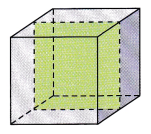STEP 2: Use a string to slice another cube diagonally, as shown. Sketch the cross section and describe its shape.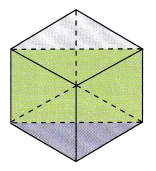STEP 3: Use a string to slice the last cube through the midpoints of each of three edges that share a common vertex, as shown. Sketch the cross section and describe its shape.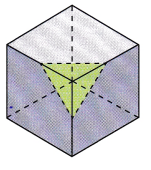Math Journal Are you able to slice a cube in other ways to form the cross-section in STEP 1 to STEP 3?

### Math in Focus Grade 7 Chapter 8 Lesson 8.1 Guided Practice Answer Key

For each solid, name the shape of the cross-section formed when the solid is sliced by the plane shown.

Question 1.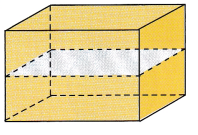Explanation: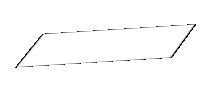The shape of the cross-section formed when the solid is sliced by the plane is a  Rectangle.
Because, It  forms a closed figure having four sides of which two of the opposite sides are equal.
So, it forms a Rectangle.

Question 2.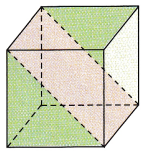Explanation: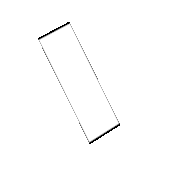The shape of the cross-section formed when the solid is sliced by the plane is a  Rectangle.
Because, It  forms a closed figure having four sides of which two of the opposite sides are equal.
So, it forms a Rectangle.

Question 3.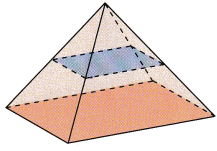Explanation: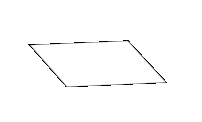The shape of the cross-section formed when the solid is sliced by the plane is a  Square.
Because, It  forms a closed figure having four sides of which all the sides are equal.
So, it forms a Square

Question 4.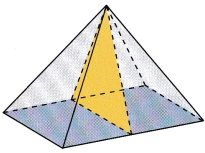Explanation: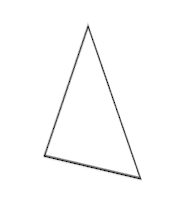The shape of the cross-section formed when the solid is sliced by the plane is a  Triangle.
Because, It  forms a closed figure having three sides .
So, it forms a Triangle.

### Math in Focus Course 2B Practice 8.1 Answer Key

Match each set to its net.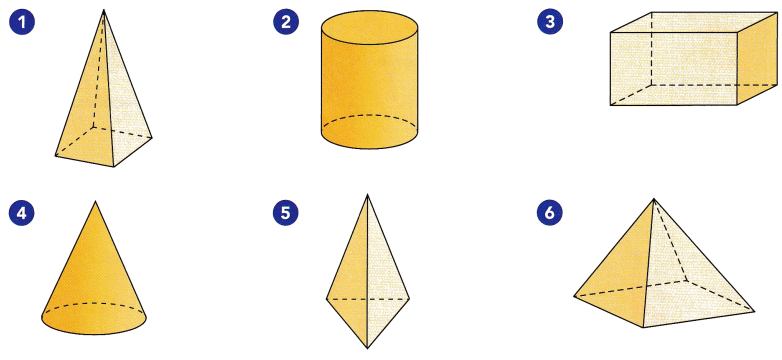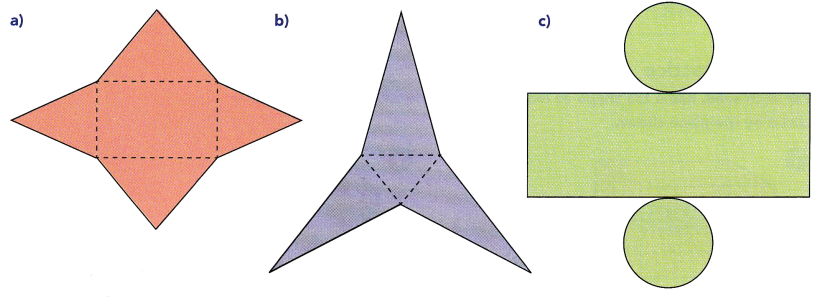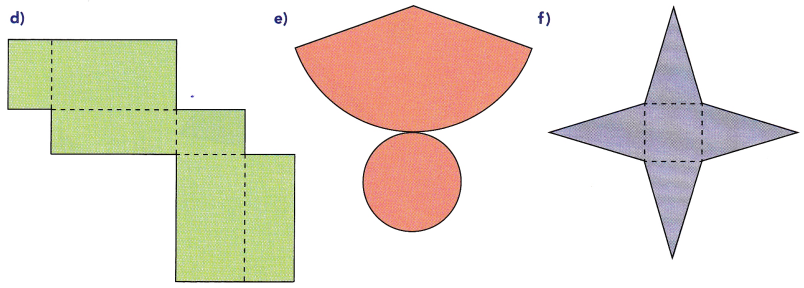Answer: The combinations of the figures are
1- f
2- c
3- d
4- e
5- b
6- a

Name the solid that can be formed from each net.

Question 7.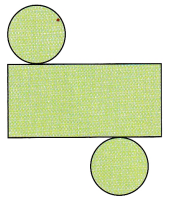Answer: The closed figure formed is a cylinder

Explanation: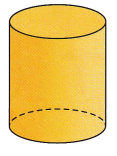It forms a cylinder

Question 8.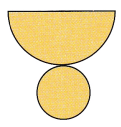Answer: The closed figure formed is a cylinder

Explanation:It is a cylinder.

Question 9.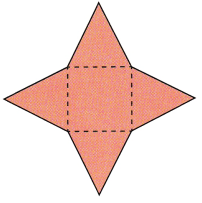Answer: The closed figure formed is a Square pyramid

Explanation: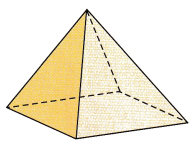It is a square pyramid

Question 10.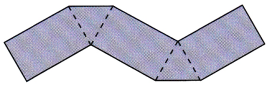Answer: The closed figure formed is a Triangular prism

Explanation: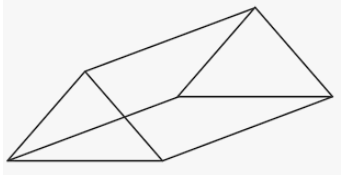It is a triangular prism.

Question 11.
Tell what cross-section is formed when a plane slices a square pyramid as described.
a) Perpendicular to its base and passes through its vertex.
Answer: The figure Perpendicular to its base and passes through its vertex is a triangle

Explanation:
Thee square pyramid is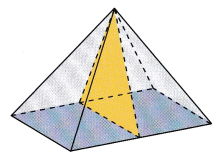So, The figure Perpendicular to its base and passes through its vertex is a triangle

b) Parallel to its base.
Answer: The figure parallel to its base is a square

Explanation:

The square pyramid is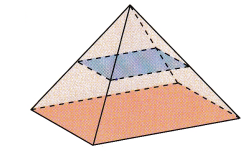So, The figure parallel to its base is a square.

Question 12.
The diagram shows a cone and its net.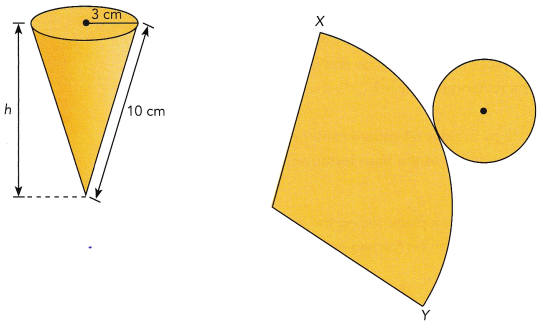a) Copy the net of the cone and label these dimensions on the net.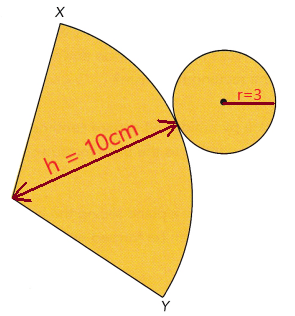b) How is the circumference of the base of the cone related to the curve XV?
Answer: The  closed figure  of the net forms a cone and its is a circle

Explanation: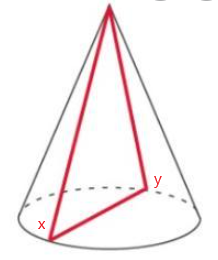Given,, the radius of a circle is 3 cm
From that the circumference of a circle is 2πr
C = 2 (3.14)(3) =  18.84 cm
So,  The xy becomes the circumference of a circle .

Question 13.
The diagram shows the net of a cylinder. Which sides of the rectangle have the same length as the circumference of the circular base?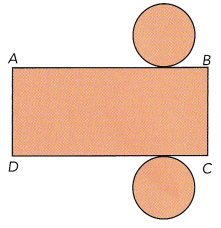Answer: The sides of the rectangle have the same length as the circumference of the circular base are  AB and DC

Explanation:
The closed figure of this  net becomes a cylinderSo, The sides of the rectangle have the same length as the circumference of the circular base are  AB and DC.

Question 14.
A base of each of the following prism is shaded. Name the shape of the cross section formed when each prism is sliced by a plane parallel to each base. Copy each prism. Sketch the cross sections and label them with the dimensions.
a)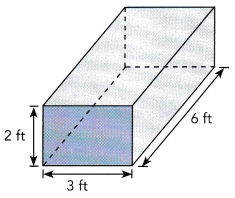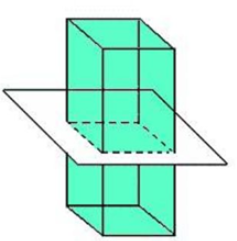b)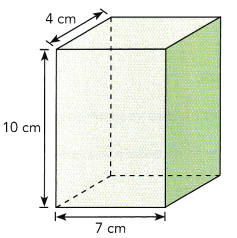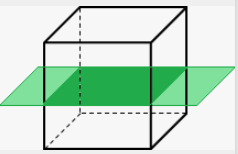Question 15.
A cross-section that is parallel to one of the bases of a rectangular prism is 3 inches wide and 6 inches long. A cross-section that is perpendicular to its bases and parallel to two other faces is 4 inches wide and 6 inches long. What are the dimensions of the rectangular prism?
Answer: The dimensions of a rectangular prism are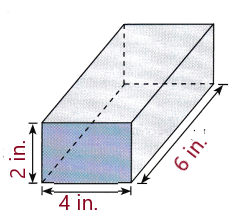Question 16.
The area of the base of a square pyramid is 64 square centimeters. Several planes slice through the pyramid parallel to the base to form square cross-sections.
a) Besides the cross-section formed by a plane slicing the base, how many cross-sections parallel to the base can be formed with areas that are perfect squares?
Answer: There can be 7 perfect squares after the multiple cross section.

Explanation:
The area of the base of a square pyramid is 64 square centimeters
That is a = 8 , Area a² = 8 × 8 = 64
So, cross-sections parallel to the base can be formed with areas that are perfect squares are
If a = 7 ,  a² = 7 × 7 = 49,
If a = 6 ,  a² = 6 × 6 = 36,
If a = 5 ,  a² = 5 × 5 = 25,
If a = 4 ,  a² = 4 × 4 = 16,
If a = 3 ,  a² = 3 × 3 = 9,
If a = 2 ,  a² = 2 × 2 = 4,
If a =  ,  a² = 1 × 1 = 1,
There can be 7 perfect squares after the multiple cross section.

b) Find the sum of the area of the base and the areas of the cross-sections found in a).
Answer: The sum of the area of the base is 28 cm²
The sum of the area of the cross-sections is  140 cm²